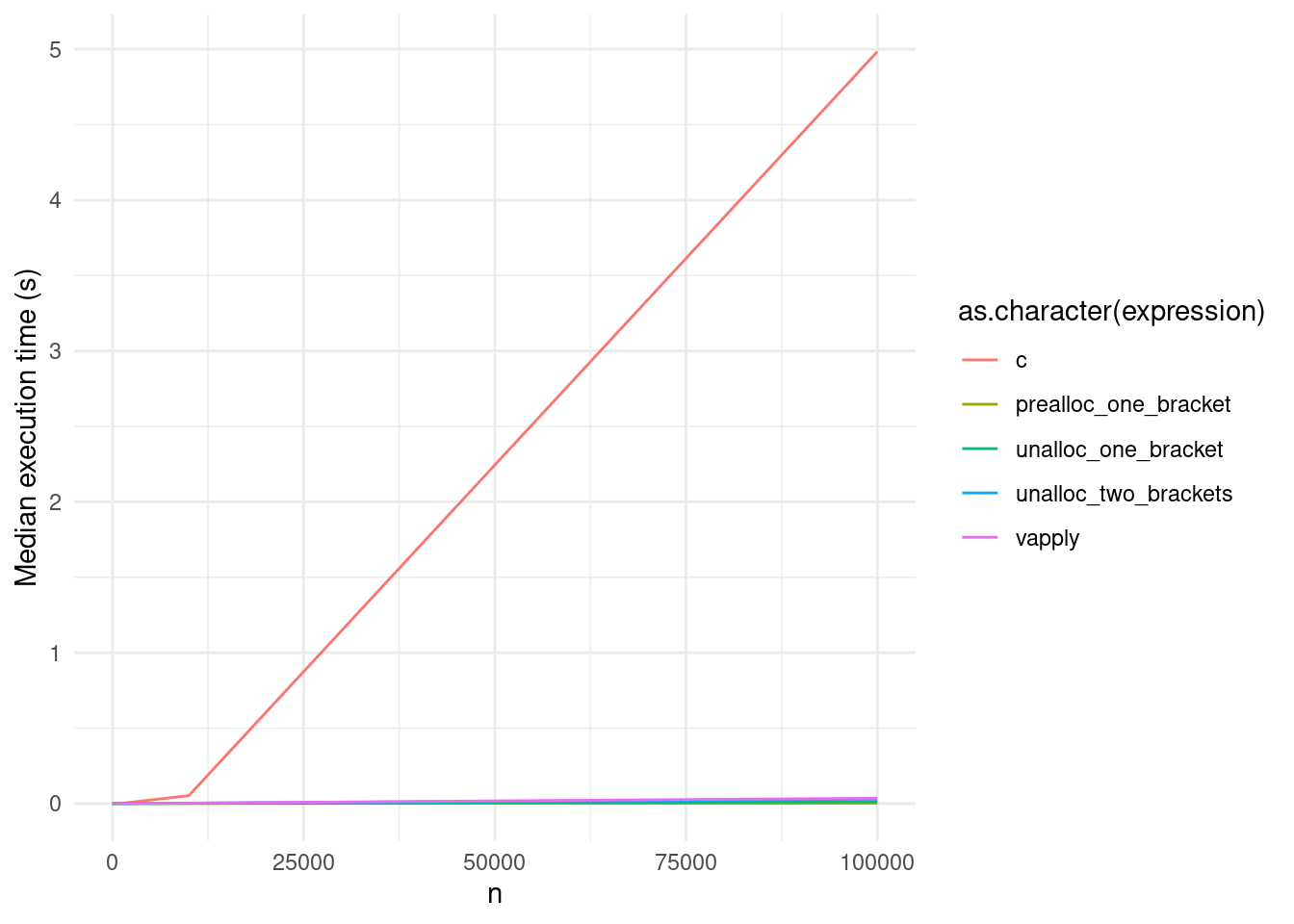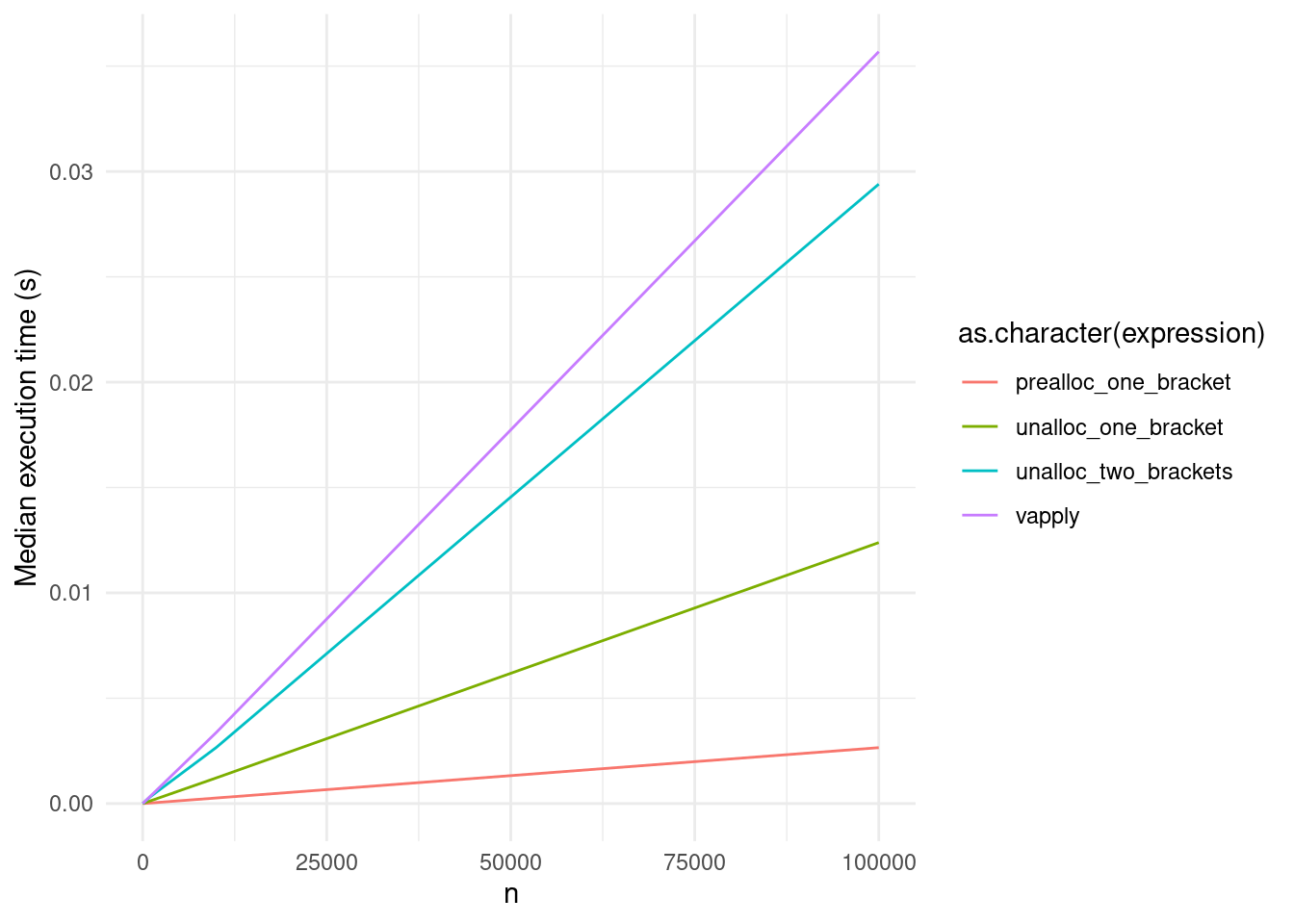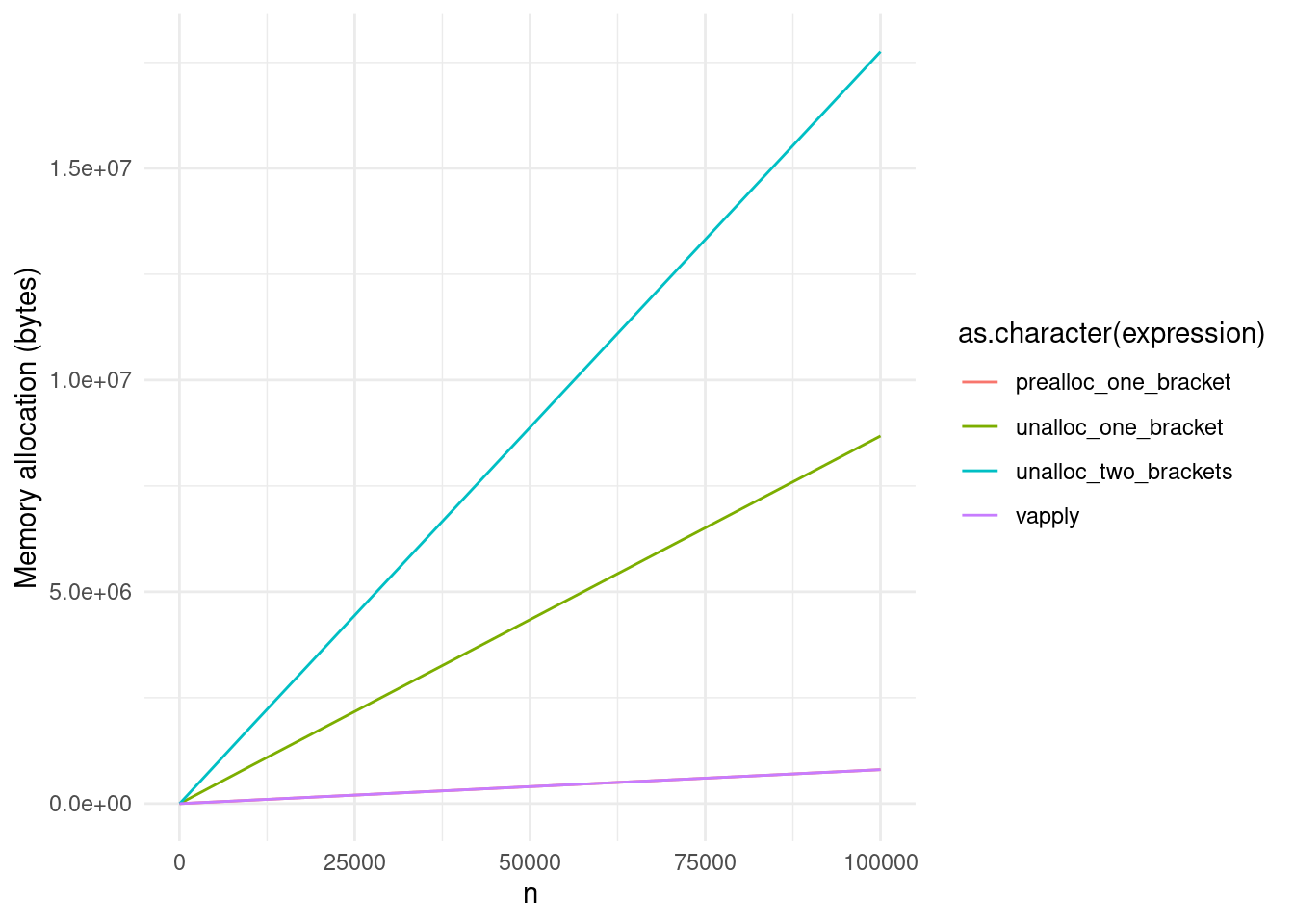# Pre-allocating vectors is for nerds

Or rather: growing objects is inefficient. But it’s maybe not as big a deal as I’d believed.
R
Tutorials
Author
Published

August 29, 2023

The second circle of R hell, in Patrick Burns’ seminal book The R Inferno, is titled “Growing Objects”. This refers to a common antipattern for R users, usually among the first things taught when dealing with iteration: it is extremely inefficient to grow a vector using `c()`, like so:

``````vector_c <- function(n) {
out <- c()
for (i in 1:n) {
out <- c(out, i)
}
out
}``````

Instead, Burns says, it is better to pre-allocate our vector `out`, and assign our function’s output to a specific position in `out` using either `[` or `[[`:

``````vector_prealloc_one_bracket <- function(n) {
out <- vector("numeric", n)
for (i in 1:n) {
out[i] <- i
}
out
}

vector_prealloc_two_bracket <- function(n) {
out <- vector("numeric", n)
for (i in 1:n) {
out[[i]] <- i
}
out
}``````

Of course, it would be better yet to avoid our loop entirely, and simply create our final object using the colon operator:

``````colon_operator <- function(n) {
1:n
}``````

But that’s beside the point right now.

This advice was originally written in 2011, but is even more important today. In Burns’ book, subsetting is roughly 7 times faster when `n` is 10,000; on my computer today, subsetting is roughly 200 times faster:

``````n <- 10000
bench::mark(
c = vector_c(n),
one_bracket = vector_prealloc_one_bracket(n),
two_brackets = vector_prealloc_two_bracket(n),
colon = colon_operator(n),
filter_gc = FALSE
)[c("expression", "median", "itr/sec", "mem_alloc")]``````
``````# A tibble: 4 × 4
expression     median `itr/sec` mem_alloc
<bch:expr>   <bch:tm>     <dbl> <bch:byt>
1 c              57.8ms      17.1   191.2MB
2 one_bracket   270.9µs    3596.     99.1KB
3 two_brackets  271.2µs    3570.     96.7KB
4 colon         371.1ns 1841212.         0B``````

But what if `n` is unknowable? Well, to quote Burns:

Often a reasonable upper bound on the size of the final object is known. If so, then create the object with that size and then remove the extra values at the end. If the final size is a mystery, then you can still follow the same scheme, but allow for periodic growth of the object.

This is still probably a decent approach: over-allocate and trim down, or allocate in chunks and only grow when those chunks are exhausted.

Or… perhaps we might try growing a vector with `[` or `[[`, rather than with `c()`? To anyone raised on R traditions, this might seem like a code smell:

``````vector_unalloc_one_bracket <- function(n) {
out <- c()
for (i in 1:n) {
out[i] <- i
}
out
}

vector_unalloc_two_bracket <- function(n) {
out <- c()
for (i in 1:n) {
out[[i]] <- i
}
unlist(out)
}``````

But if we test it out:1

``````bench::mark(
c = vector_c(n),
prealloc_one_bracket = vector_prealloc_one_bracket(n),
unalloc_one_bracket = vector_unalloc_one_bracket(n),
unalloc_two_brackets = vector_unalloc_two_bracket(n),
filter_gc = FALSE
)[c("expression", "median", "itr/sec", "mem_alloc")]``````
``````# A tibble: 4 × 4
expression             median `itr/sec` mem_alloc
<bch:expr>           <bch:tm>     <dbl> <bch:byt>
1 c                     61.23ms      14.8  191.23MB
2 prealloc_one_bracket 269.86µs    3632.    78.17KB
3 unalloc_one_bracket    1.27ms     679.   871.73KB
4 unalloc_two_brackets   2.86ms     316.     1.72MB``````

Growing a vector via `[` is still notably slower than assigning values to a pre-allocated vector; it looks like it’s roughly ~5 times slower. But that still means it’s ~50 times faster than growing a vector via `c()`, and allocates ~200 times less memory to do so. Growing a vector via `[[` isn’t quite as efficient – taking roughly twice the time and memory as `[` here – but still blows `c()` out of the water.

That’s not too shabby, for a code smell. How does a method like `vapply()` compare?

``````vapply_lambda <- function(n) {
vapply(1:n, \(i) i, numeric(1))
}

bench::mark(
c = vector_c(n),
prealloc_one_bracket = vector_prealloc_one_bracket(n),
unalloc_one_bracket = vector_unalloc_one_bracket(n),
unalloc_two_brackets = vector_unalloc_two_bracket(n),
vapply = vapply_lambda(n),
filter_gc = FALSE
)[c("expression", "median", "itr/sec", "mem_alloc")]``````
``````# A tibble: 5 × 4
expression             median `itr/sec` mem_alloc
<bch:expr>           <bch:tm>     <dbl> <bch:byt>
1 c                     51.14ms      19.2   191.2MB
2 prealloc_one_bracket 268.72µs    3643.     78.2KB
3 unalloc_one_bracket    1.33ms     567.      853KB
4 unalloc_two_brackets   2.72ms     341.      1.7MB
5 vapply                 3.44ms     270.     78.2KB``````

`vapply()` uses as little memory as our pre-allocation approaches, but is slower than either of our un-allocated methods.2

It’s worth emphasizing that the differences between these methods are microscopic compared to the difference between them and `c()` for growing vectors:

``````benchmarks <- bench::press(
bench::mark(
c = vector_c(n),
prealloc_one_bracket = vector_prealloc_one_bracket(n),
unalloc_one_bracket = vector_unalloc_one_bracket(n),
unalloc_two_brackets = vector_unalloc_two_bracket(n),
vapply = vapply_lambda(n),
filter_gc = FALSE
),
n = c(10, 100, 1000, 10000, 100000)
)

library(ggplot2)
ggplot(benchmarks, aes(n, median, color = as.character(expression))) +
geom_line() +
theme_minimal() +
labs(y = "Median execution time (s)")``````But as far as execution speed goes, well, maybe growing objects in general isn’t worthy of its own circle of hell anymore:

``````benchmarks[as.character(benchmarks\$expression) != "c", ] |>
ggplot(aes(n, median, color = as.character(expression))) +
geom_line() +
theme_minimal() +
labs(y = "Median execution time (s)")``````Though of course, `vapply()` and the pre-allocated methods still win out in terms of memory allocation:3

``````benchmarks[as.character(benchmarks\$expression) != "c", ] |>
ggplot(aes(n, mem_alloc, color = as.character(expression))) +
geom_line() +
theme_minimal() +
labs(y = "Memory allocation (bytes)")``````So: pre-allocate your vectors when you’re able. But maybe it’s fine to grow an object every once in a while, as a treat. It probably won’t get you sent to hell.

I have no idea when things changed to make growing vectors via `[` so much more efficient now than in 2011 – and please let me know in the comments/Mastodon/BlueSky if you know any more details here.

## Footnotes

1. I dropped `prealloc_two_brackets` from the benchmarks because it was performing ~the same as the one-bracket alternative.↩︎

2. Usual disclaimer that this is probably not a type of slowness that matters for your code, that you should look into moving computation to C++/Rust if you care about a few milliseconds execution time, and that the real benefits of *apply functions come from readability and their potential for parallelization, not speed.↩︎

3. The pre-allocated line is hidden by the `vapply()` line; they’re practically identical, and possibly also literally identical.↩︎